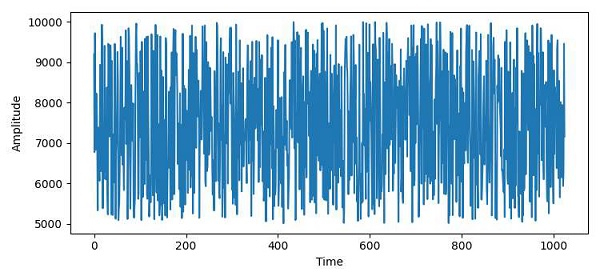# How to plot a wav file using Matplotlib?

To plot a .wav file using matplotlib, we can take following the steps −

• To read a .wav file, we can use the read() method.

• After reading the .wav file, we will get a tuple. At the 0 th index, rate would be there and at the 1st index, array sample data.

• Use the plot() method to plot the .wav file.

• Set y and x labels using ylabel and xlabel with “Amplitude” and “Time” label, respectively.

• To display the figure, use the show() method.

## Example

from scipy.io.wavfile import read
import matplotlib.pyplot as plt
plt.rcParams["figure.figsize"] = [7.50, 3.50]
plt.rcParams["figure.autolayout"] = True
plt.show()